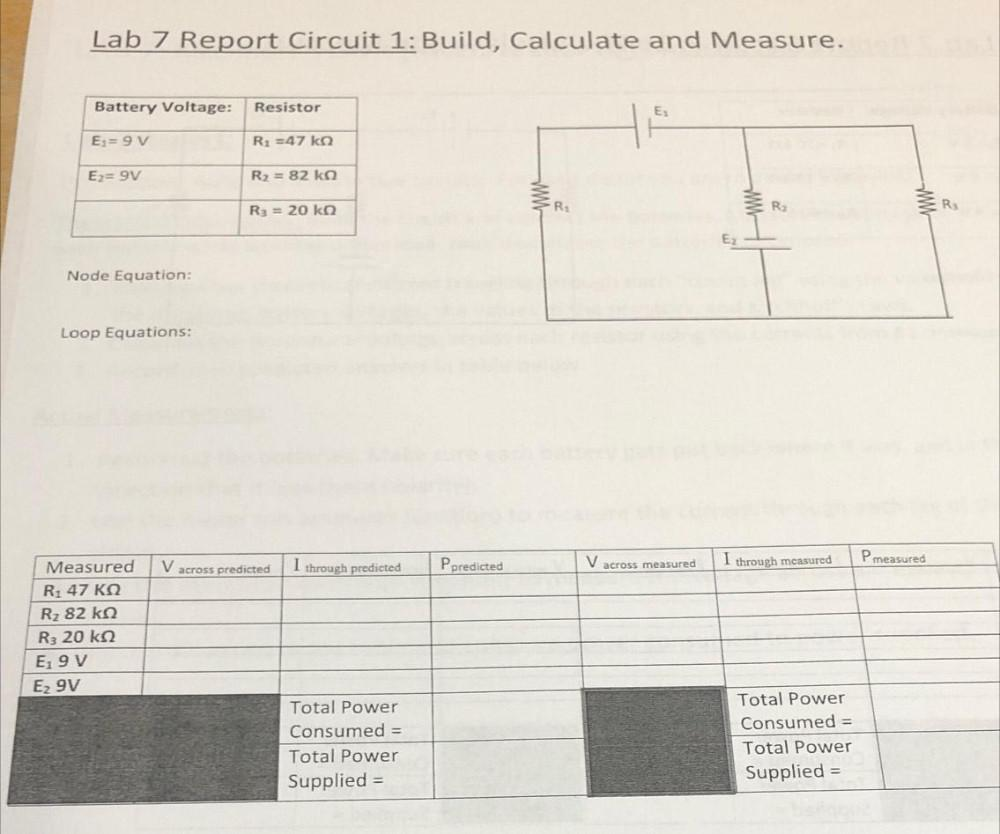Question:

# Lab 7 Report Circuit 1: Build, Calculate and Measure. Battery Voltage: Resistor E: E= 9V R2 =47ko Ez= 9V R2 = 82 ka R3 = 20 ko RLab 7 Report Circuit 1: Build, Calculate and Measure. Battery Voltage: Resistor E: E= 9V R2 =47ko Ez= 9V R2 = 82 ka R3 = 20 ko R: R Rs Node Equation: Loop Equations: V across predicted P measured I through predicted Ppredicted V across measured I through measured Measured R1 47 KO Rz 82 ke R3 20 k2 Egy E2 9V Total Power Consumed = Total Power Supplied = Total Power Consumed = Total Power Supplied =